Supply - Theory of Demand and Supply

# Supply - Theory of Demand and Supply Notes | Study Business Economics for CA Foundation - CA Foundation

## Document Description: Supply - Theory of Demand and Supply for CA Foundation 2022 is part of Business Economics for CA Foundation preparation. The notes and questions for Supply - Theory of Demand and Supply have been prepared according to the CA Foundation exam syllabus. Information about Supply - Theory of Demand and Supply covers topics like and Supply - Theory of Demand and Supply Example, for CA Foundation 2022 Exam. Find important definitions, questions, notes, meanings, examples, exercises and tests below for Supply - Theory of Demand and Supply.

Introduction of Supply - Theory of Demand and Supply in English is available as part of our Business Economics for CA Foundation for CA Foundation & Supply - Theory of Demand and Supply in Hindi for Business Economics for CA Foundation course. Download more important topics related with notes, lectures and mock test series for CA Foundation Exam by signing up for free. CA Foundation: Supply - Theory of Demand and Supply Notes | Study Business Economics for CA Foundation - CA Foundation
 1 Crore+ students have signed up on EduRev. Have you?

LEARNING OUTCOMES
At the end of this Unit, you should be able to:

• Explain the meaning of supply.
• List and provide specific examples of determinants of supply.
• Describe the law of supply.
• Describe the difference between movements on the supply curve and shift of the supply curve.
• Explain the concept of elasticity of supply with examples.
• Illustrate how the concepts of demand and supply can be used to determine price.

INTRODUCTION
In a market economy, sellers for products and services constitute the supply side. As the term ‘demand’ refers to the quantity of a good or service that the consumers are willing and able to purchase at various prices during a given period of time, the term ‘supply’ refers to the amount of a good or service that the producers are willing and able to oer to the market at various prices during a given period of time.
Two important points apply to supply:
(i) Supply refers to what a firm offer for sale in the market, not necessarily to what they succeed in selling. What is offered may not get sold.
(ii) Supply is a flow. The quantity supplied is ‘so much’ per unit of time, per day, per week, or per year.
DETERMINANTS OF SUPPLY
Although price is an important consideration in determining the willingness and desire to part with commodities, there are many other factors which determine the supply of a product or a service. These are discussed below:
(i) Price of the good: Other things being equal, the higher the relative price of a good the greater the quantity of it that will be supplied. This is because goods and services are produced by the firm in order to earn profits and, ceteris paribus, profits rise if the price of its product rises.
(ii) Prices of related goods: If the prices of other goods rise, they become relatively more profitable to the firm to produce and sell than the good in question. It implies that, if the price of Y rises, the quantity supplied of X will fall. For example, if price of wheat rises, the farmers may shift their land to wheat production away from corn and soya beans.
(iii) Prices of factors of production: Cost of production is a significant factor that affects supply. A rise in the price of a particular factor of production will cause an increase in the cost of making those goods that use a great deal of that factor than in the costs of producing those that use relatively small amount of the factor. For example, a rise in the cost of land will have a large effect on the cost of producing wheat and a very small effect on the cost of producing automobiles. Thus, a change in the price of one factor of production will cause changes in the relative profitability of different lines of production and will cause producers to shift from one line to another and thus supplies of different commodities will change.
(iv) State of technology: The supply of a particular product depends upon the state of technology also. Inventions and innovations tend to make it possible to produce more or better goods with the same resources, and thus they tend to increase the quantity supplied of some products and to reduce the quantity supplied of products that are displaced. Availability of spare production capacity and the ease with which factor substitution can be made and the cost of such substitution also determine supply.
(v) Government Policy: The production of a good may be subject to the imposition of commodity taxes such as excise duty, sales tax and import duties. These raise the cost of production and so the quantity supplied of a good would increase only when its price in the market rises. Subsidies, on the other hand, reduce the cost of production and thus provide an incentive to the firm to increase supply. When government imposes restrictions such as import quota on inputs, rationing of input supply etc, production tends to fall.
(vi) Nature of competition and size of industry: Under competitive conditions, supply will be more than that under monopolized conditions. If there are large number of firms in the market, supply will be more. Besides, entry of new firms, either domestic or foreign, causes the industry supply curve to shift rightwards.
Other Factors: The quantity supplied of a good also depends upon government’s industrial and foreign policies, goals of the firm, infrastructural facilities, natural factors such as weather, floods, earthquake and man-made factors such as war, labour strikes, communal riots and etc.

LAW OF SUPPLY
This refers to the relationship of quantity supplied of a good with one or more related variables which have an influence on the supply of the good. Normally, supply is related with price but it can be related with the type of technology used, scale of operations etc. The law of supply can be stated as: Other things remaining constant, the quantity of a good produced and offered for sale will increase as the price of the good rises and decrease as the price falls.
This law is based upon common sense, because the higher the price of the good, the greater the profits that can be earned and thus greater the incentives to produce the good and offer it for sale. The law is known to be correct in a large number of cases. There is an exception however. If we take the supply of labour at very high wages, we may find that the supply of labour has decreased instead of increasing. Thus, the behaviour of supply depends upon the phenomenon considered and the degree of possible adjustment in supply.
The behaviour of supply is also affected by the time taken into consideration. In the short run, it may not be easy to increase supply, but in the long run supply can be easily adjusted in response to changes in price.
The law of supply can be explained through a supply schedule and a supply curve. A supply schedule is the tabular presentation of the law of supply. It shows the different prices of a commodity and the corresponding quantities that suppliers are willing to offer for sale. Consider the following hypothetical supply schedule of good X.

Table 9 : Supply Schedule of Good ‘X’

 Price (₹) (per kg) Quantity supplied (kg) 1 5 2 35 3 45 4 55 5 65

The table shows the quantities of good X that would be produced and offered for sale at a number of alternative prices. At ₹ 1, for example, 5 kilograms of good X are offered for sale and at ₹ 3 per kg. 45 kg. would be forthcoming.
We can now plot the data from Table 9 on a graph. In Figure 19, price is plotted on the vertical axis and quantity on the horizontal axis, and various price-quantity combinations of the schedule 9 are plotted.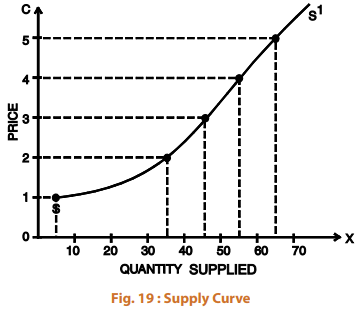When we draw a smooth curve through the plotted points, what we get is the supply curve for good X. The supply curve is a graphical presentation of the supply schedule. It shows the quantity of X that will be offered for sale at each price of X. It slopes upwards towards right (positive slope) showing that as price increases, the quantity supplied of X increases and vice-versa.
The market supply, like market demand, is the sum of supplies of a commodity made by all individual firms or their supply agencies. The market supply is governed by the law of supply. The market supply curve for ‘X’ can be obtained by adding horizontally the supply curves of various firms.
MOVEMENTS ON THE SUPPLY CURVE – INCREASE OR DECREASE IN THE QUANTITY SUPPLIED
When the supply of a good increase as a result of an increase in its price, we say that there is an increase in the quantity supplied and there is a upward movement on the supply curve. The reverse is the case when there is a fall in the price of the good. (See Figure 21)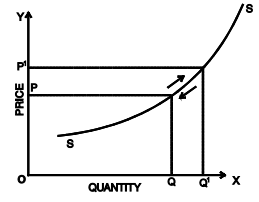Fig. 20: Figure showing change in quantity supplied as a result of a price change
SHIFTS IN SUPPLY CURVE – INCREASE OR DECREASE IN SUPPLY
When the supply curve bodily shifts towards the right as a result of a change in one of the factors that influence the quantity supplied other than the commodity’s own price, we say there is an increase in supply. When these factors cause the supply curve to shift to the left, we call it decrease in supply [See Figures 21(i) and (ii)].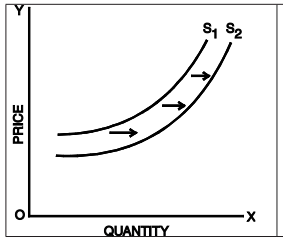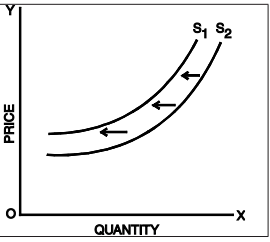Fig. 21 : Shifts in supply curves

ELASTICITY OF SUPPLY
The elasticity of supply is defined as the responsiveness of the quantity supplied of a good to a change in its price. Elasticity of supply is measured by dividing the percentage change in quantity supplied of a good by the percentage change in its price i.e.,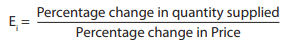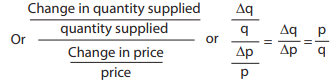Where
q   denotes original quantity supplied.
Δq   denotes change in quantity supplied.
p   denotes original price.
Δp   denotes change in price.
Example:
a) Suppose the price of commodity X increases from ₹ 2,000 per unit to ₹ 2,100 per unit and consequently the quantity supplied rises from 2,500 units to 3,000 units. Calculate the elasticity of supply.
Here ∆q = 500 units         ∆p = ₹ 100
p = ₹ 2000           q = 2500 units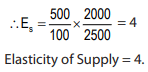Type of Supply Elasticity
The elasticity of supply can be classified as under:
(i) Perfectly inelastic supply: If as a result of a change in price, the quantity supplied of a good remains unchanged, we say that the elasticity of supply is zero or the good has perfectly inelastic supply. The vertical supply curve in Figure 22 shows that irrespective of price change, the quantity supplied remains unchanged.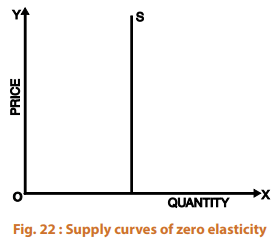(ii) Relatively less-elastic supply: If as a result of a change in the price of a good its supply changes less than proportionately, we say that the supply of the good is relatively less elastic or elasticity of supply is less than one. Figure 23 shows that the relative change in the quantity supplied (∆q) is less than the relative change in the price (∆p).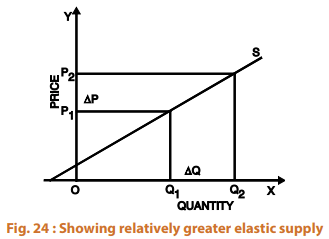(iv) Unit-elastic: If the relative change in the quantity supplied is exactly equal to the relative change in the price, the supply is said to be unitary elastic. Here the coefficient of elasticity of supply is equal to one. In Figure 25, the relative change in the quantity supplied (∆q) is equal to the relative change in the price (∆p)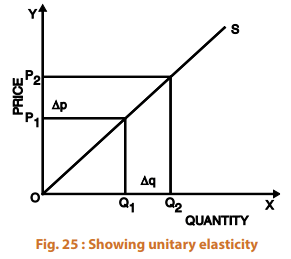(v) Perfectly elastic supply: Elasticity of supply said to be infinite when nothing is supplied at a lower price, but a small increase in price causes supply to rise from zero to an infinitely large amount indicating that producers will supply any quantity demanded at that price. Figure 26 shows infinitely elastic supply.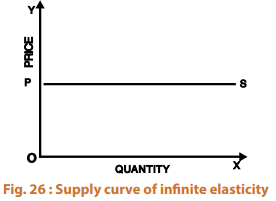Measurement of supply-elasticity
The elasticity of supply can be considered with reference to a given point on the supply curve or between two points on the supply curve. When elasticity is measured at a given point on the supply curve, it is called point elasticity.Just as in demand, point-elasticity of supply can be measured with the help of the following formula: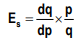Qus: The Supply function is given as q = -100 + 10p. Find the elasticity of supply using point method, when price is ₹ 15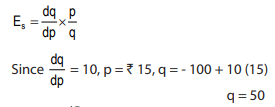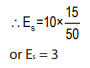Where dq/dp is differentiation of the supply function with respect to price and p and q refer to price and quantity respectively .
Arc-Elasticity: Arc-elasticity i.e. elasticity of supply between two prices can be found out with the help of the following formula: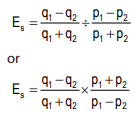Where p1 q1 are original price and quantity and p2 q2 are new price and quantity supplied. Thus, if we have to find elasticity of supply when p1 = ₹ 12, p2 = ₹ 15, q1 = 20 units and q2 = 50 units. Then using the above formula, we will get supply elasticity as: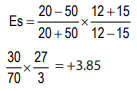Equilibrium Price
Equilibrium refers to a market situation where quantity demanded is equal to quantity supplied. The intersection of demand and supply determines the equilibrium price. At this price the amount that the buyers want to buy is equal to the amount that sellers want to sell. Only at the equilibrium price, both the buyers and sellers are satisfied. Equilibrium price is also called market clearing price.
The determination of market price is the central theme of micro economic analysis. Hence, micro-economic theory is also called price theory.
The following table explains the equilibrium price
Table 10 : Supply and Demand Schedule

 Price (₹) Quantity Demanded Quantity Supplied Impact on price 5 6 31 Downward 4 12 25 Downward 3 19 19 Equilibrium 2 25 12 Upward 1 21 6 Upward

The equilibrium between demand and supply is depicted in the diagram below. Demand and supply are in equilibrium at point E where the two curves intersect each other. It means that only at price ₹ 3 the quantity demanded is equal to the quantity supplied. The equilibrium quantity is 19 units and these are exchanged at price ₹ 3. If the price is more than the equilibrium level, excess supply will push the price downwards as there are few takers in the market at this price. For example, in Table 10, if price is say ₹ 5, quantity demanded is 6 units which is quite less than the quantity supplied (31 units). There will be excess supply in the market which will force the sellers to reduce price if they want to sell off their product. Hence the price will fall and continue falling down till the level where quantity demanded becomes equal to the quantity supplied. Opposite will happen when quantity demanded is more than quantity supplied at a particular price.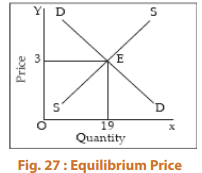The equilibrium price is determined by the intersection between demand and supply. It is also called the market equilibrium.

 SUMMARY“Supply’ refers to the amount of a good or service that the producers are willing and able to oer to the market at various prices during a given period of time.The determinants of supply other than its own price are: prices of the related goods, prices of factors of production, state of technology, government policy and other factors.The law of supply states that when the price of the good rises, the corresponding quantity supplied increases and when the price reduces, the quantity supplied also reduces. There is a direct relationship between price and quantity supplied.The supply curve establishes the relationship between the amount of supply and the price. It is an upward sloping curve showing a positive relationship between price and quantity supplied.When the supply of a good increases as a result of an increase in its price we say that there is an increase in the quantity supplied and there is an upward movement on the supply curve. The reverse is the case when there is a fall in the price of the good.Elasticity of supply means the responsiveness of supply to change in the price of the commodity.The elasticity of supply can be classified in to perfectly inelastic supply, relatively less-elastic supply, relatively greater-elastic supply, unit-elastic and perfectly elastic supply.The measurement of supply-elasticity is of two types- point elasticity and arc-elasticity.Elasticity of supply can be considered with reference to a given point on the supply curve (point elasticity) or between two points on the supply curve (arc elasticity).Equilibrium price is one at which the wishes of both the buyers and the sellers are satisfied. At this price, the amount that buyers want to buy and sellers want to sell will be equal.
The document Supply - Theory of Demand and Supply Notes | Study Business Economics for CA Foundation - CA Foundation is a part of the CA Foundation Course Business Economics for CA Foundation.
All you need of CA Foundation at this link: CA Foundation

## Business Economics for CA Foundation

61 videos|111 docs|67 tests
 Use Code STAYHOME200 and get INR 200 additional OFF

## Business Economics for CA Foundation

61 videos|111 docs|67 tests

Track your progress, build streaks, highlight & save important lessons and more!

,

,

,

,

,

,

,

,

,

,

,

,

,

,

,

,

,

,

,

,

,

;